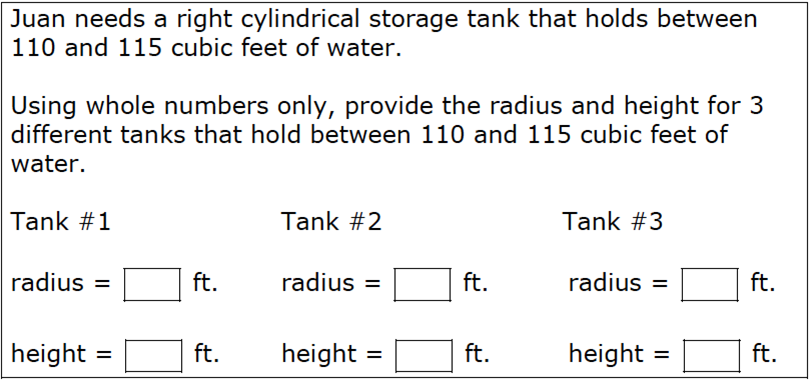# Cylinders### Hint

Is there a formula that can help us solve this problem?

When it says, “the tank holds between 110 and 115 cubic feet of water,” what mathematical concept are they referring to?

r=1 and h = 36, r = 2 and h = 9, r = 3 and h = 4, r = 6 and h = 1

## Interior and Exterior Angles of Triangles

Directions: In triangle ABC, angle ABC is obtuse. Using the digits 1 to 9 at …

1.Also, (1 , 36 )= (r , h )

•2.3.4.